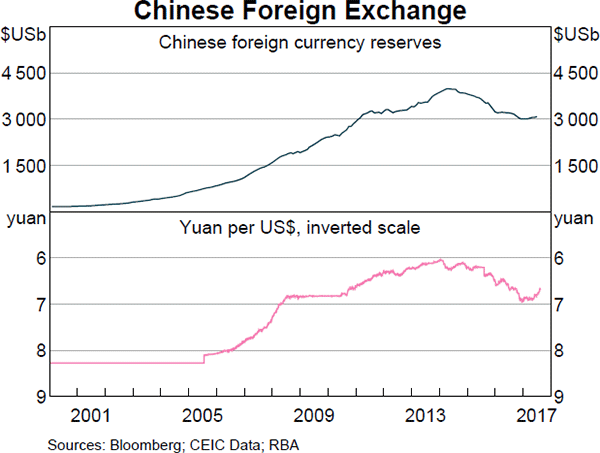# Fight Finance

#### CoursesTagsRandomAllRecentScores

For a price of $13, Carla will sell you a share which will pay a dividend of$1 in one year and every year after that forever. The required return of the stock is 10% pa.

Would you like to Carla's share or politely ?

Question 65  annuity with growth, needs refinement

Which of the below formulas gives the present value of an annuity with growth?

Hint: The equation of a perpetuity without growth is: $$V_\text{0, perp without growth} = \frac{C_\text{1}}{r}$$

The formula for the present value of an annuity without growth is derived from the formula for a perpetuity without growth.

The idea is than an annuity with T payments from t=1 to T inclusive is equivalent to a perpetuity starting at t=1 with fixed positive cash flows, plus a perpetuity starting T periods later (t=T+1) with fixed negative cash flows. The positive and negative cash flows after time period T cancel each other out, leaving the positive cash flows between t=1 to T, which is the annuity.

\begin{aligned} V_\text{0, annuity} &= V_\text{0, perp without growth from t=1} - V_\text{0, perp without growth from t=T+1} \\ &= \dfrac{C_\text{1}}{r} - \dfrac{ \left( \dfrac{C_\text{T+1}}{r} \right) }{(1+r)^T} \\ &= \dfrac{C_\text{1}}{r} - \dfrac{ \left( \dfrac{C_\text{1}}{r} \right) }{(1+r)^T} \\ &= \dfrac{C_\text{1}}{r}\left(1 - \dfrac{1}{(1+r)^T}\right) \\ \end{aligned}

The equation of a perpetuity with growth is:

$$V_\text{0, perp with growth} = \dfrac{C_\text{1}}{r-g}$$

In these tough economic times, central banks around the world have cut interest rates so low that they are practically zero. In some countries, government bond yields are also very close to zero.

A three year government bond with a face value of 100 and a coupon rate of 2% pa paid semi-annually was just issued at a yield of 0%. What is the price of the bond? One formula for calculating a levered firm's free cash flow (FFCF, or CFFA) is to use net operating profit after tax (NOPAT). \begin{aligned} FFCF &= NOPAT + Depr - CapEx -\Delta NWC \\ &= (Rev - COGS - Depr - FC)(1-t_c) + Depr - CapEx -\Delta NWC \\ \end{aligned} \\ Does this annual FFCF or the annual interest tax shield? One year ago you bought100,000 of shares partly funded using a margin loan. The margin loan size was $70,000 and the other$30,000 was your own wealth or 'equity' in the share assets.

The interest rate on the margin loan was 7.84% pa.

Over the year, the shares produced a dividend yield of 4% pa and a capital gain of 5% pa.

What was the total return on your wealth? Ignore taxes, assume that all cash flows (interest payments and dividends) were paid and received at the end of the year, and all rates above are effective annual rates.

Hint: Remember that wealth in this context is your equity (E) in the house asset (V = D+E) which is funded by the loan (D) and your deposit or equity (E).

A pig farmer in the US is worried about the price of hogs falling and wants to lock in a price now. In one year the pig farmer intends to sell 1,000,000 pounds of hogs. Luckily, one year CME lean hog futures expire on the exact day that he wishes to sell his pigs. The futures have a notional principal of 40,000 pounds (about 18 metric tons) and currently trade at a price of 63.85 cents per pound. The underlying lean hogs spot price is 77.15 cents per pound. The correlation between the futures price and the underlying hogs price is one and the standard deviations are both 4 cents per pound. The initial margin is USD1,500 and the maintenance margin is USD1,200 per futures contract.

Which of the below statements is NOT correct?

Here is a table of stock prices and returns. Which of the statements below the table is NOT correct?

 Price and Return Population Statistics Time Prices LGDR GDR NDR 0 100 1 99 -0.010050 0.990000 -0.010000 2 180.40 0.600057 1.822222 0.822222 3 112.73 0.470181 0.624889 0.375111 Arithmetic average 0.0399 1.1457 0.1457 Arithmetic standard deviation 0.4384 0.5011 0.5011

A put option written on a risky non-dividend paying stock will mature in one month. As is normal, assume that the option's exercise price is non-zero and positive $(K>0)$ and the stock has limited liability $(S>0)$.

Which of the following statements is NOT correct? The put option's:

On 1 February 2016 you were told that your refinery company will need to purchase oil on 1 July 2016. You were afraid of the oil price rising between now and then so you bought some August 2016 futures contracts on 1 February 2016 to hedge against changes in the oil price. On 1 February 2016 the oil price was $40 and the August 2016 futures price was$43.

It's now 1 July 2016 and oil price is $45 and the August 2016 futures price is$46. You bought the spot oil and closed out your futures position on 1 July 2016.

What was the effective price paid for the oil, taking into account basis risk? All spot and futures oil prices quoted above and below are per barrel.

The Chinese central bank has the largest amount of foreign currency reserves.What could the large amounts of foreign exchange reserves held by the Chinese government be used for in a currency crisis? China's currency is called the Renminbi (RMB) or Yuan (CNY). In a Chinese currency crisis the Chinese government is likely to use its FX reserves to: